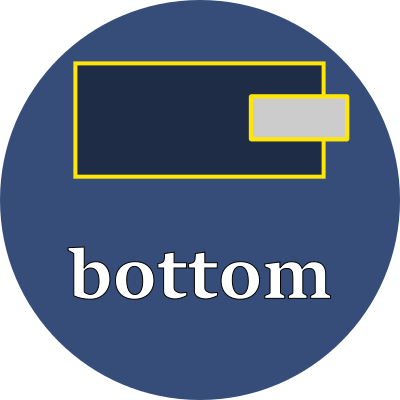bottomExplicitly set the bottom edge of the element.

Property values

This property has different interpretations, depending upon the element's position property.

position interpretation
static This property is ignored.
relative Shifts the normal flow-of-text position by the given amount. Positive values shift it up from the bottom. Negative values shift it outside the element's defined bottom boundary.
fixed Sets the bottom edge of the element to be the given amount above the bottom edge of the browser's viewport.
absolute Sets the bottom edge of the element to be the given amount above the bottom edge of the ancestor's viewport, but only if that ancestor has a position that is relative, fixed or absolute.
sticky Follows the rules for relative until the bottom value is scrolled to. After that, follows the rules for fixed and uses the given value as a fixed amount from the browser's bottom edge.

Units

Use any of these font-relative typographic units to set the bottom:

 em em The font-size of the current font rem relative em The font-size of the element lh line height The line-height of the current font rlh relative line height The line-height of the element cap cap height The nominal height of the current font's capital letters ex "x" The height of the current font's "x" glyph

Or use any of these fixed size units which are independent of the current font:

 % percent A percentage of the parent element's height in inch A size equal 96px px pixels A size equal to 1/96 inch pt point A size equal 1in/72 = 1.33px pc pica A size equal 1in/6 = 12pt = 16px cm centimeter A size equal to 96px/2.54 = 37.8px mm millimeter A size equal to 96px/25.4 = 3.8px Q quarter millimeter A size equal to 96px/1016 = 0.94px

Examples

style > position > bottomBottom edge of element